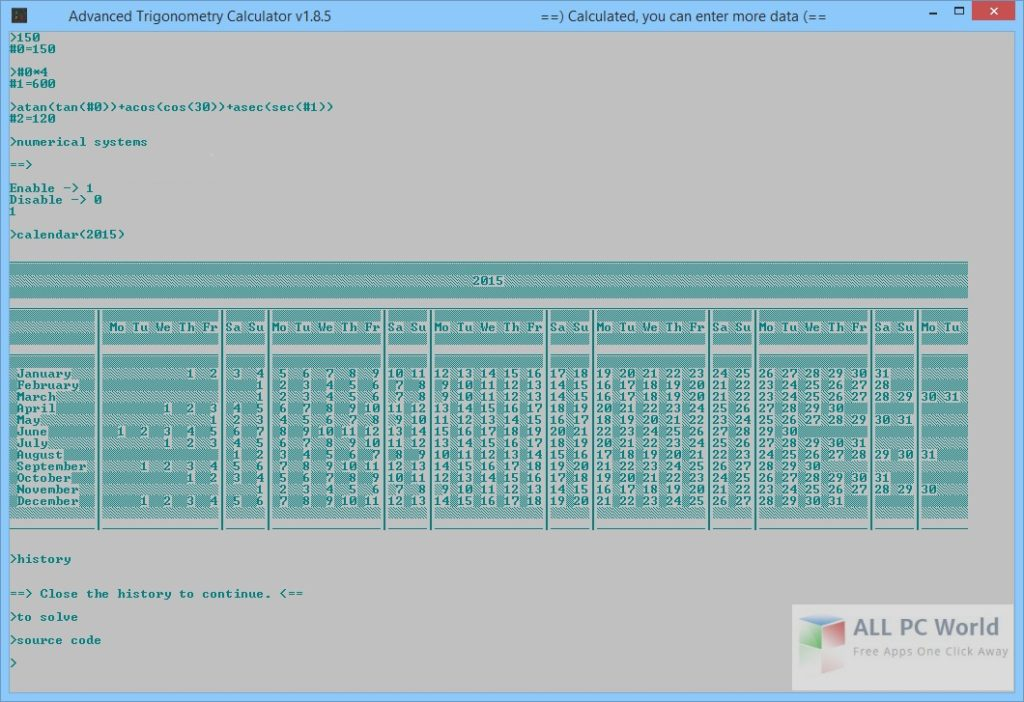Advanced Trigonometry Calculator Portable 2.0 is a calculation tool for mathematical functions, it is a portable tool with a command-based interface with the capability to handle multiple expressions at the same time. This free download is the standalone portable version of Advanced Trigonometry Calculator for Windows 32-bit and 64-bit.

## Advanced Trigonometry Calculator Portable 2.0 Overview

There are very few tools for handling mathematical expressions, Advanced Trigonometry Calculator is an open source portable calculator which does not require to install. You can carry it anywhere with you in any removable storage and run on any computer without leaving any traces after removal. It can figure out any trigonometrical expression along with support for calculating multiple expressions at the same time. The command-based interface is friendly and requires little technical information. A detailed help manual is provided for the new users, with all the available commands.This powerful tool can support all trigonometric functions like sin, cos, tan in degrees, radians, and gradians, along with hyperbolic expressions (cosh, sinh, tanh), digital signal processing, arithmetic (addition, subtraction, quotient, multiplication), logarithmic expression, quadratic equations, statistical data, and more. Moreover, you can add long expressions with parenthesis. It is a lightweight tool which can not consume much of your system resources.

## Features of Advanced Trigonometry Calculator Portable 2.0

Some of the features of Advanced Trigonometry Calculator are:

• Lightweight and user-friendly tool
• Command-based mathematical calculations
• Open-source application
• Detailed help manual
• Calculate multiple expressions at the same time
• Supports all trigonometric functions
• Digital Signal Processing
• Arithematic and logarithmic expressions
• Calculate quadratic equations and statistical data
• Expressions reusability
• Maintains history
• Identifies errors and much more